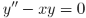# Triple factorials and Airy functions

Last week I wrote in a post on multifactorials in which I said that

Double factorials come up fairly often, and sometimes triple, quadruple, or higher multifactorials do too.

This post gives a couple examples of triple factorials in practice.

One example I wrote about last week. Triple factorial comes up when evaluating the gamma function at an integer plus 1/3. As shown here,Another example involves solutions to Airy’s differential equationOne pair of independent solutions consists of the Airy functions Ai(x) and Bi(x). Another pair consists of the functionsgiven in A&S 10.4.3. Because both pairs of functions solve the same linear ODE, each is a linear combination of the other.

Notice that the numerators are triple factorials, and so the series above can be rewritten asThe next post gives an example of quadruple factorials in action.